# Finding the Equation of a Parabola from the Focus and Directrix

An error occurred trying to load this video.

Try refreshing the page, or contact customer support.

Coming up next: Foci and the Definitions of Ellipses and Hyperbolas

### You're on a roll. Keep up the good work!

Replay
Your next lesson will play in 10 seconds
• 0:00 Parabola Curves
• 2:07 Parabola Equation in…
• 3:23 Parabola Equation in…
• 4:19 Parabola Equation in…
• 5:41 Lesson Summary
Save Save

Want to watch this again later?

Timeline
Autoplay
Autoplay
Speed Speed Audio mode

#### Recommended Lessons and Courses for You

Lesson Transcript
Instructor: Gerald Lemay

Gerald has taught engineering, math and science and has a doctorate in electrical engineering.

A parabola is the familiar shape seen in many physical applications, like the path taken by a ball thrown upwards. This lesson explores equations for the parabola and shows how they may be obtained from two quantities: the focus and the directrix.

## Parabola Curves

Remember the last time you played with blocks? Great fun! With lettered blocks, you can build words. It is amazing so much variety exists with only 26 letters. Also, the shapes of letters themselves are inspirational. For example, a parabola is shaped like the letter U.In this lesson, learn to build various equations for the parabola given only the information contained in the focus and the directrix. Now those are words we probably did not see with our blocks.

Start by drawing a line on a graph. In this example, the line goes through y = -3 and is parallel to the x-axis. This line is called the directrix. Locate a point. In this example, the point is at (3,1). This point is called the focus.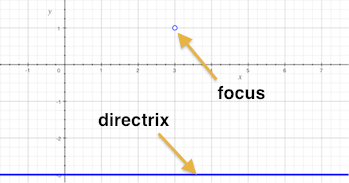The idea is to use only this information to plot a parabola and to find equations that define the parabola. The parabola corresponding to this directrix and focus looks like this: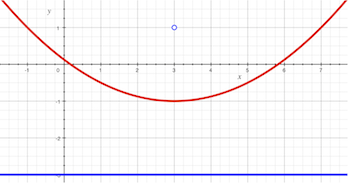The distance from the focus to a point on the parabola curve is the same as the shortest distance from this point on the parabola curve to the directrix line. So, the points on the parabola curve are equidistant (equal distance) to the focus and to the directrix. Finding all the equidistant points is one way to plot the parabola. The blue lines on the image below show the equal lengths.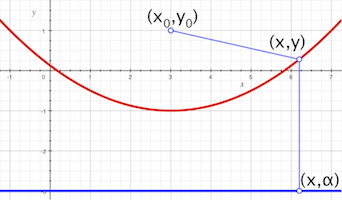The turning point on the parabola is the vertex. On this example, the vertex is at (3, -1), which we observe to be halfway between the focus and the directrix. Lots of words to build with our blocks.

Instead of building words, this is building equations. Say the focus is here: (xo,yo). The point on the line is (x,y). The location on the directrix will be at (x,α). Let's keep things general on purpose. In addition to the variables x and y, the equations will have your three numbers xo, yo and α.

## Parabola Equation in Standard Form

The parabola equation in standard form for finding the length between two points is the square root of the sum of the squared differences in each coordinate. For this case, the length of the line segment between the focus at (xo,yo) and the point on the curve (x,y) is the square root of (xo - x)^2 + (yo - y)^2. The length of the line segment between the point on the curve (x,y) and the point on the directrix (x,α) is the square root of (x - x)^2 + (y - α)^2. The lengths are equal so you can equate the two square roots. Then, square both sides, expand, and isolate the y on the left-hand side of the equation.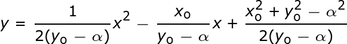The right-hand side depends on xo, yo, and α. This equation has the same form as y = ax^2 + bx + c. This is the standard form equation for the parabola. Do you see how to find 'a', 'b', and 'c'?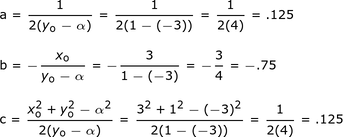Our example parabola in standard form is y = .125x^2 - .75x + .125.

To unlock this lesson you must be a Study.com Member.

### Register to view this lesson

Are you a student or a teacher?

#### See for yourself why 30 million people use Study.com

##### Become a Study.com member and start learning now.
Back
What teachers are saying about Study.com

### Earning College Credit

Did you know… We have over 200 college courses that prepare you to earn credit by exam that is accepted by over 1,500 colleges and universities. You can test out of the first two years of college and save thousands off your degree. Anyone can earn credit-by-exam regardless of age or education level.